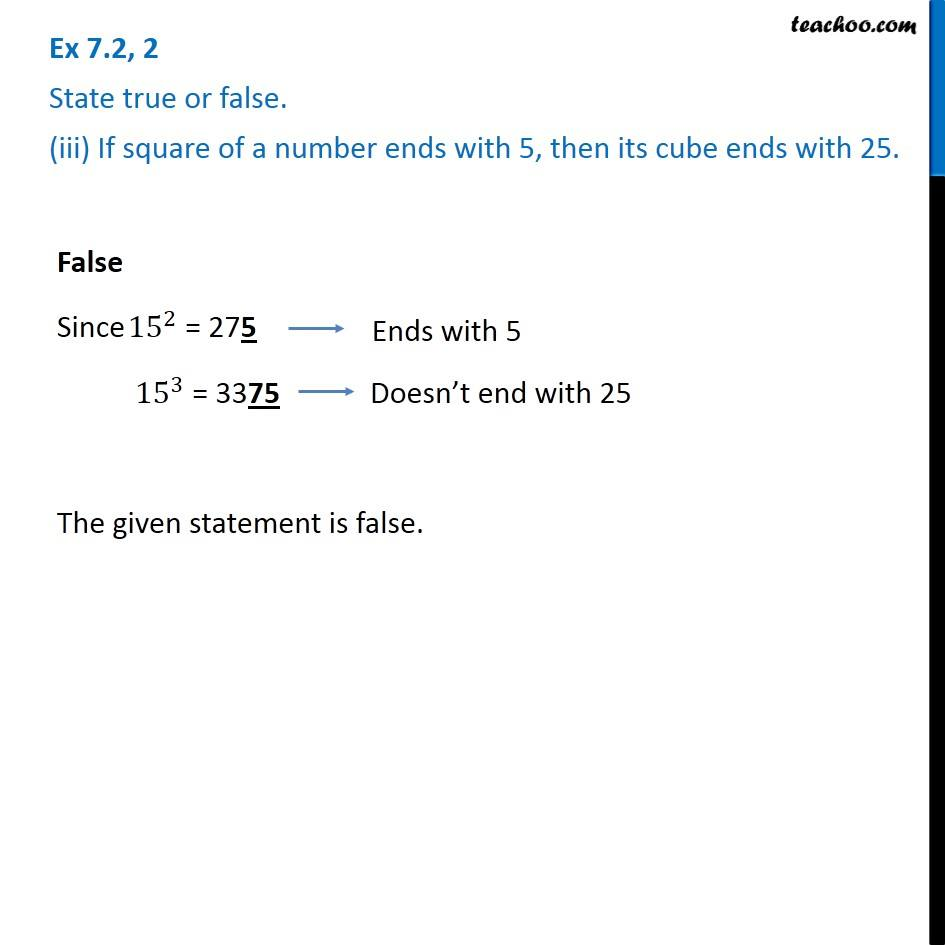1. Chapter 7 Class 8 Cubes and Cube Roots
2. Serial order wise
3. Ex 7.2

Transcript

Ex 7.2, 2 State true or false. (iii) If square of a number ends with 5, then its cube ends with 25.False Since 〖15〗^2 = 275 〖15〗^3 = 3375 The given statement is false.

Ex 7.2

Chapter 7 Class 8 Cubes and Cube Roots
Serial order wise